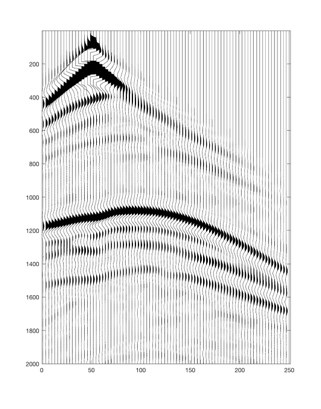# Computational Geoscience Group

## Inverse Problems and Large-scale Models

An Inverse problems is, in its simplest form, a problem of data-fitting. We seek a parameterized model of a physical (or biological, or economical) system, that is able to 'explain' observed data within the measurement errors. Examples are:

• Computing CO2 emission and absorption over a geographical area from observations in a network of measurement stations, using numerical simulations of atmospheric flow
• Computing the infection mechanism of a virus from test data
• Computing parameters of an economic model from statistical data about consumer behavior
• Computing parameters of the earth (e.g., seismic wave propagation velocities) from seismic data, using the wave equation

Data fitting is an optimization problem (the difference between observed data and data computed from the system model must be "small"), but in an inverse problem there are at least two complications:

1. The data fitting problem often does not have a unique solution. So, how do we choose a reasonable solution (out of the many)?
2. Observational data are almost always contaminated by noise, and this will make any solution uncertain. How do we compute this uncertainty?

Inverse problem theory provides ways of tackling the above challenges, but current research is focused on an additional problem: Many real-life problems cannot be solved because there are too many unknown parameters and the computation of data from the system parameters is computationally expensive. How can the search for feasible solutions be significantly improved? The answer to this question is probably to incorporate additional information about the problem in the solution algorithm. But how can this be done?

### Using approximate physics models to solve large-scale inverse problemsPreliminary investigations indicate that guiding the search for solutions to an inverse problem using approximations to the full physical relation between unknown model parameters and data, could dramatically improve the computational speed, even without reducing the accuracy of the solution. If confirmed, this approach would be a breakthrough in inverse theory. A possible project would be to develop a general formulation and algorithm for this type of method.

Contact: Klaus Mosegaard.

### Using empirical (learning-based) information to solve large-scale inverse problemsRecent successes in application of Machine Learning (ML) to a range of problems have raised the question: How can ML be used in solution of inverse problems? Shall we only use it to replace the 'forward function' that maps model parameters into data? Or shall we try to compute solutions directly from data using ML? How do we deal with observational noise in an algorithm based on ML? What does ML do to the inherent non-uniquenes of solutions? All these questions are at the cutting edge of current research, and is a possible topic for a project.

Contact: Klaus Mosegaard.